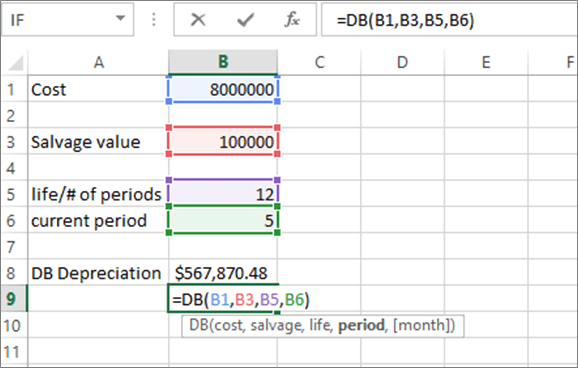# Excel Formulas for Accounting and Finance

Excel spreadsheet is a common tool used by every accountant or financial analyst to analyze, report, and share financial information. Some basic excel functions let you perform complex calculations and produce useful models making your work more effective and easier. Excel can become a more versatile tool if you increase your knowledge on more other financial analysis functions.

In this article, I will focus on some of the most common accounting and financial formulas you can use for basic to complex calculations.

1. XNPV

The function is used to determine the company's worth using the Net Present Value of a series of discounted cash flows. Unlike the Excel NPV, the XNPV function uses specific dates for cash flows

Syntax

=XNPV (discount_rate, cash_flows, dates)2. XIRR

XIRR determines the internal rate of return for a series of cash flows with specific dates. The XIRR should always be used over IRR if the time periods are different.

Syntax

=XIRR (cash flows, dates, [estimated_irr])

The estimated_irr parameter is optional. It is your guess at the internal rate of return. If the parameter is omitted, it assumes a constant value of 10%.Excel NPV and IRR assume time periods in the cash flow are equal but there are times where the cash flows are not timed evenly therefore XNPV and XIRR are used to solve the problem.

3. MIRR

This function is very important in analyzing the internal rate of return. It is a modified formula used when cash from one investment is used in a different investment. If the cash for new land is invested in buying a new motor vehicle.

Syntax

=MIRR (cash flows, cost of borrowing, reinvestment rate)

Assuming a company produces an internal rate of return, IRR with a finance rate of 6%, and the cash is reinvested in a motor vehicle at a rate of 5%, then MIRR will be higher than the IRR rate.4. PMT

PMT function is used in real estate, commercial banking, or in financial analysis of positions with debt schedules. The formula is mostly used as a mortgage payment calculator. Once the interest rate is provided, the time period, and the available mortgage loan, you can easily calculate the payment amount.

Syntax

=PMT (rate, number of time periods, present value)5. IPMT

IPMT calculates the interest rate of the fixed debt payments. The function works in conjunction with the PMT function. To get the principal payment for each period, you can separate the interest payments for each period and make the difference between PMT and IPMT.

Syntax

=IPMT (rate, current period#, total #of periods, present value)

Example: The interest payment in year 4 is (\$4.762.75) on a 30-year loan payment period with an interest rate of 5%.6. EFFECT

This function is important in lending and borrowing businesses. It returns the annual interest rate for non-annual compounding.

Syntax

= EFFECT (interest rate, #of periods per year)7. FV

This function helps you to predict how much cash you will have in the future, given the starting amount, the regular amount payments, and the compounding interest rate.

Syntax

=FV (rate, # of periods, payments, starting value, type)8. DB

This function is used by financial professionals to calculate depreciation expenses. This enables them to avoid building a large Declining Balance.

Syntax

=DB (cost, salvage value, life/# of periods, current period)9. RATE

The rate function can calculate the Yield to maturity for given security. It is commonly used when determining the average annual return earned from buying bonds.

Syntax

=RATE (# of periods, coupon payment per period, price of bond, face value of bond, type)10. ACCRINT

This function returns accrued interest for a security that pays periodic interest.

Syntax:

=ACCRINT (issue_date, first_interest_date, settlement_date, rate, par, frequency, [basis], [calc_method])

11. AMORDEGRC:

Returns depreciation for each accounting period by using a depreciation coefficient.

Syntax

=AMORDEGRC (cost, purchase_date, first_period, salvage, period, rate, [basis])12. Amorlinc:

Returns the depreciation of assets for each accounting period.

Syntax

= AMORLINC (cost, purchase_date, first_period, salvage, period, rate, [basis])13. DDB

This function returns the depreciation of an asset for a given period based on the double-declining method.

Syntax

=DDB (cost, salvage, life, period, [factor])14. SYD

SYD function returns the depreciation of an asset for a given time period based on the sum of years of the depreciation method.

Syntax

=SYD (cost, salvage, life, period)